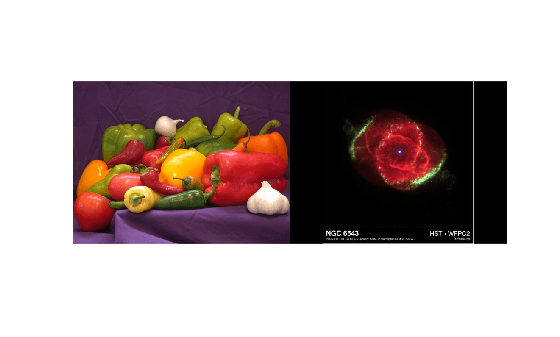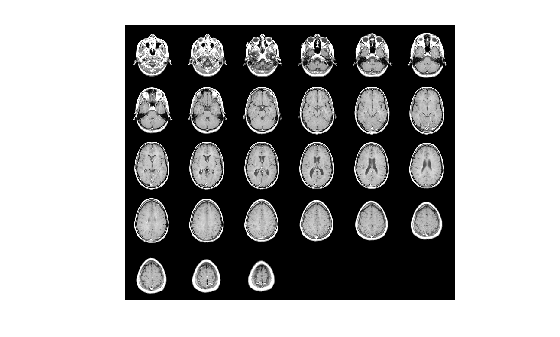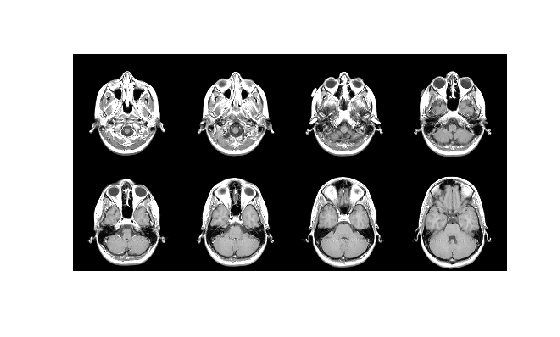imtile

Combine multiple image frames into one rectangular tiled image

Description

out = imtile(filenames) returns a tiled image containing the images in the files with file names filenames.

By default, the imtile function arranges the images so that they roughly form a square. You can change the arrangement using optional name-value arguments. The images can have different sizes and data types.

• If you specify an indexed image, then the imtile function converts it to RGB using the colormap present in the file.

• If there is a data type mismatch between images, then the imtile function converts all images to data type double using the im2double function.

out = imtile(I) returns a tiled image containing all the frames of the multiframe image array I. A multiframe image array can be a sequence of binary, grayscale, or truecolor images.

out = imtile(images) returns a tiled image containing the images specified in the cell array images. imtile displays empty cell array elements as a blank tile.

out = imtile(imds) returns a tiled image containing the images specified in the ImageDatastore object imds. For information about image datastores, see ImageDatastore.

out = imtile(___,map) returns a tiled image in which grayscale, indexed, and binary images are converted to RGB using the colormap map. You can specify input images using the input argument of any of the preceding syntaxes. Note that if you specify images using file names filenames, and also specify the colormap, then map overrides any internal colormap present in the image files.

out = imtile(___,Name,Value) returns a customized tiled image, depending on the values of the optional parameter name-value pair arguments.

Examples

collapse all

Read multiple images from files into the workspace and create a tiled image containing the images. Display the tiled image.

out = imtile({'peppers.png', 'ngc6543a.jpg'});
imshow(out);Using a data set containing multiple images, tile the images in a grid.

out = imtile(D, map);
imshow(out);Create a tiled image containing only the first eight images in the data set. Use the 'GridSize' parameter to arrange the images in a 2-by-4 grid.

out = imtile(D, map, 'Frames', 1:8, 'GridSize', [2 4]);
figure;
imshow(out);Read an RGB image into the workspace.

Create a tiled image containing each of the three planes of the RGB image. Display the tiled image.

out = imtile(imRGB);
imshow(out)From an image datastore, create and customize a tiled image.

Create an image datastore containing all the files with the file extension 'tif' or 'png' in the specified folder.

fileFolder = fullfile(matlabroot,'toolbox','matlab','imagesci');
imds = imageDatastore(fileFolder,'FileExtensions',{'.tif','.png'});

Create a tiled image containing the images in the datastore.

out1 = imtile(imds);
imshow(out1);Use the 'BorderSize' and 'BackgroundColor' parameters to add a blue border to the tiled image.

out2 = imtile(imds, 'BorderSize', 10, 'BackgroundColor', 'b');
figure;
imshow(out2);Input Arguments

collapse all

Names of files containing images, specified as an f-by-1 string array, a 1-by-f string array, a character vector, or a cell array of character vectors. If the files are not in the current folder or in a folder on the MATLAB® path, then specify the full path name. For more information, see imread.

Example: 'file1.jpg'

Example: ["file1.jpg" "file2.jpg"]

Example: '../dir/data/file1.png'

Example: {'C:\dir\data\file1.tif','C:\dir\data\file2.tif'}

Data Types: char | string | cell

Multiframe image, specified as a numeric array. I can be:

• An m-by-n-by-k array representing a sequence of k binary, grayscale, or indexed images

• An m-by-n-by-1-by-k array representing a sequence of k binary, grayscale, or indexed images

• An m-by-n-by-3-by-k array representing a sequence of k truecolor images

List of images, specified as an k-by-1 or 1-by-k cell array of numeric matrices. The cell array can contain numeric matrices of size m-by-n or m-by-n-by-3.

Image datastore, specified as an ImageDatastore object.

Colormap, specified as a c-by-3 numeric matrix with values in the range [0,1]. Each row of map is a three-element RGB triplet that specifies the red, green, and blue components of a single color. When you specify map, the imtile function converts all indexed, grayscale, and binary images to truecolor using this colormap.

Data Types: double

Name-Value Arguments

Specify optional comma-separated pairs of Name,Value arguments. Name is the argument name and Value is the corresponding value. Name must appear inside quotes. You can specify several name and value pair arguments in any order as Name1,Value1,...,NameN,ValueN.

Example: imtile({'peppers.png','ngc6543a.jpg'},'BackgroundColor','g');

Color of the background, specified as an RGB triplet, a color name, or a short color name. The imtile function fills all blank spaces with this color, including the space specified by 'BorderSize'.

You can specify any color using an RGB triplet. An RGB triplet is a 3-element row vector whose elements specify the intensities of the red, green, and blue components of the color. The intensities must be in the range [0,1].

You can specify some common colors by name as a string scalar or character vector. This table lists the named color options and the equivalent RGB triplets.

Color NameShort NameRGB TripletAppearance
'red''r'[1 0 0]'green''g'[0 1 0]'blue''b'[0 0 1]'cyan' 'c'[0 1 1]'magenta''m'[1 0 1]'yellow''y'[1 1 0]'black''k'[0 0 0]'white''w'[1 1 1]Example: 'BackgroundColor','r'

Example: 'BackgroundColor','green'

Example: 'BackgroundColor',[0 0.4470 0.7410]

Padding around each thumbnail image, specified as the comma-separated pair consisting of 'BorderSize' and a numeric scalar or 1-by-2 numeric vector of the form [brows bcols]. The imtile function pads the borders of each image with the background color.

Frames to include, specified as the comma-separated pair consisting of 'Frames' and a numeric array or a logical mask. The imtile function interprets the values as indices into the image array or cell array. The following examples create a tiled image containing the first three image frames.

Example: out = imtile(I,'Frames',1:3);

Example: out = imtile(I,'Frames',[true true true]);

Number of rows and columns of thumbnails in the tiled image, specified as the comma-separated pair consisting of 'GridSize' and a two-element vector of the form [nrows ncols]. nrows specifies the number of rows in the grid and ncols specifies the number of columns in the grid. Use NaNs or Infs to have imtile calculate the size in a particular dimension in a way that includes all the images.

• If 'GridSize' is [2 NaN], then imtile creates a tiled image with two rows and the number of columns necessary to include all the images.

• If both the elements are NaN or Inf, then imtile calculates the grid size to form a square. imtile returns the images horizontally across columns.

• If there is a mismatch between GridSize and number of images (frames), then imtile creates the tiled image based on GridSize.

Size of thumbnails, specified as the comma-separated pair consisting of 'ThumbnailSize' and a two-element vector of the form [trows tcols], in pixels. The imtile function preserves the aspect ratio of the original image by zero-padding the boundary.

• If you specify a NaN or Inf, then the imtile function calculates the corresponding value automatically to preserve the aspect ratio of the first image.

• If you specify an empty array ([]), then the imtile function uses the full size of the first image as the thumbnail size.

Output Arguments

collapse all

Tiled output image, returned as a numeric matrix or a numeric array. The output image is an M-by-N-by-3 numeric array when any of these conditions are true:

• At least one of the input images is an RGB image or indexed image

• You specify a colormap, map

• You specify a background color, 'BackgroundColor', regardless of whether the tiled image includes background pixels or not